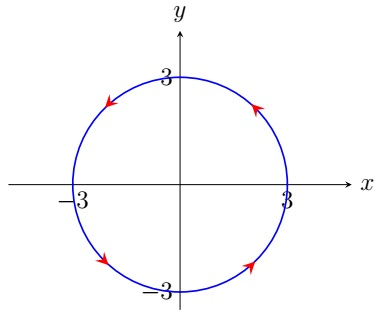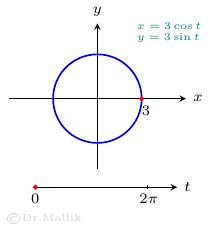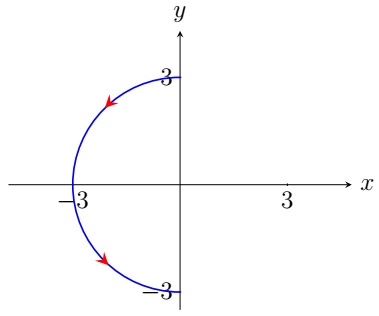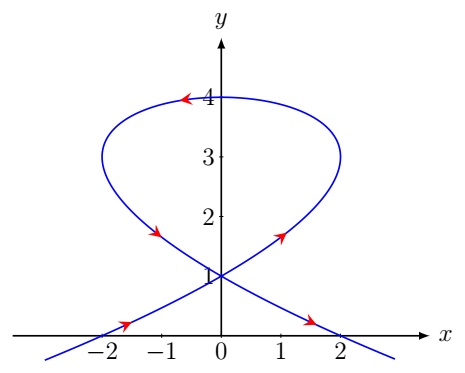Calculus II Home

## Parametric Equations

Sometimes it is impossible to write the equation of a curve in the form $$y=f(x)$$ or $$x=f(y)$$. But we may be able to write $$x$$ and $$y$$ in terms of a third variable or parameter, say $$t$$, in a set of equations called parametric equations: $x=f(t),\; y=g(t),\; a\leq t\leq b.$ A curve given by parametric equations is called a parametric curve which is sometimes written as $c(t)=(f(t),g(t)),\; a\leq t\leq b.$ Note that $$c(t)$$ is drawn with an orientation using the values of $$t$$, starting from the initial point $$(f(a),g(a))$$ and ending at the terminal point $$(f(b),g(b))$$.Example.

1. The parametric equations of the circle $$x^2+y^2=9$$ are $x=3\cos t,\; y=3\sin t,\; 0\leq t\leq 2\pi.$ It can be verified as follows: $x^2+y^2=(3\cos t)^2 +(3\sin t)^2=9(\cos^2 t+\sin^2 t)=9.$ The top and bottom halves of the circle are given by $$0\leq t\leq \pi$$ and $$\pi\leq t\leq 2\pi$$ respectively. The left semicircle of the preceding circle can be written as $x=3\cos t,\; y=3\sin t,\; \frac{\pi}{2}\leq t\leq \frac{3\pi}{2}.$ The initial point of the semicircle is $$\left(3\cos \left( \frac{\pi}{2} \right),3\sin \left( \frac{\pi}{2} \right) \right)=(0,3)$$ and the terminal point is $$\left(3\cos \left( \frac{3\pi}{2} \right),3\sin\left( \frac{3\pi}{2} \right)\right)=(0,-3)$$.2. The parametric equations of the circle $$(x-a)^2+(y-b)^2=r^2$$ are $x=a+r\cos t,\; y=b+r\sin t,\; 0\leq t\leq 2\pi.$
3. The parametric equations of the ellipse $$\displaystyle\frac{(x-\alpha)^2}{a^2}+\frac{(y-\beta)^2}{b^2}=1$$ are $x=\alpha+a\cos t,\; y=\beta+b\sin t,\; 0\leq t\leq 2\pi.$
4. The parametric equations of the line segment from $$(a_1,b_1)$$ to $$(a_2,b_2)$$ are $x=(1-t)a_1+ta_2,\; y=(1-t)b_1+tb_2,\; 0\leq t \leq 1.$
5. The parametric equations of the line $$y=mx+b$$ are $x=t,\; y=mt+b,\; -\infty < t <\infty.$ The above is a natural parametrization of the line where $$x$$ is the parameter $$t$$ and $$y$$ is a function of $$t$$.

Implicitization of Parametric Curves:
Sometimes we can eliminate the parameter $$t$$ form parametric equations $$x=f(t),\; y=g(t)$$ of a curve and write an implicit equation in $$x$$ and $$y$$.

Example. Find an equation for each of the following parametric curves: (a) $$x=t^2+2,\,y=t+1$$, $$-1\leq t < \infty$$, (b) $$c(t)=(\tan t,\sec^2 t)$$, $$-\displaystyle\frac{\pi}{2} < t < \frac{\pi}{2}$$.

Solution. (a) By solving for $$t$$ from $$y=t+1$$, we get $$t=y-1$$. Substituting $$t=y-1$$ in $$x=t^2+2$$, we get $x=(y-1)^2+2.$ Note that $$t=-1$$ corresponds to $$(3,0)$$ and $$y=t+1$$ increases with $$t$$. Thus the above parabola starts from $$(3,0)$$ and goes to the increasing direction of $$y$$.

(b) Here $$x=\tan t$$ and $$y=\sec^2 t$$. Since $$\sec^2 t-\tan^2 t=1$$, $y-x^2=1 \implies y=x^2+1.$ Since $$-\infty < \tan t < \infty$$ for $$-\displaystyle\frac{\pi}{2}< t < \frac{\pi}{2}$$, $$-\infty < x < \infty$$. Thus $$c(t)=(\tan t,\sec^2 t)$$, $$-\displaystyle\frac{\pi}{2}< t < \frac{\pi}{2}$$ represents the parabola $$y=x^2+1$$.
Note that the parabola $$y=x^2+1$$ can be parametrized in multiple ways. For example, its natural parametrization is $c(t)=(t,t^2+1),\; -\infty < t < \infty.$

Derivatives from Parametric Equations:
Consider the following parametric equations: $x=f(t),\; y=g(t),\; a\leq t\leq b,$ where $$x$$ and $$y$$ are differentiable functions of $$t$$ and $$y$$ is a differentiable function of $$x$$. Then $$y$$ is a differentiable function of $$t$$. By the chain rule, $\frac{dy}{dt}=\frac{dy}{dx} \frac{dx}{dt}$ which implies $\frac{dy}{dx}=\frac{\frac{dy}{dt}}{\frac{dx}{dt}}=\frac{y'(t)}{x'(t)},\; \text{ provided } x'(t)\neq 0.$$$c(t)=(t^3-3t,4-t^2)$$

Example. Find the equations of the tangent lines to the parametric curve $$c(t)=(t^3-3t,4-t^2)$$ at $$(0,1)$$.

Solution. First note that $$t^3-3t=t(t^2-3)=0 \implies t=0,\pm \sqrt{3}$$. Since $$t=0\implies y=4-t^2=4$$ and $$t=\pm \sqrt{3}\implies y=4-t^2=1$$, both $$t=\sqrt{3}$$ and $$t=-\sqrt{3}$$ correspond to $$(0,1)$$ where $$c(t)=(t^3-3t,4-t^2)$$ self-intersects. $\frac{dy}{dx}=\frac{y'(t)}{x'(t)} =\frac{-2t}{3t^2-3}.$ The slope of the tangent line corresponding to $$t=\sqrt{3}$$ is $\frac{dy}{dx}=\frac{y'(t)}{x'(t)} =\left. \frac{-2t}{3(t^2-1)} \right\vert_{t=\sqrt{3}}=\frac{-\sqrt{3}}{3}.$ Thus the equation of the tangent line corresponding to $$t=\sqrt{3}$$ is $y-1=\frac{-\sqrt{3}}{3}x.$ Similarly the slope of the tangent line corresponding to $$t=-\sqrt{3}$$ is $\frac{dy}{dx}=\frac{y'(t)}{x'(t)} =\left. \frac{-2t}{3(t^2-1)} \right\vert_{t=-\sqrt{3}}=\frac{\sqrt{3}}{3}$ and the equation of the tangent line corresponding to $$t=-\sqrt{3}$$ is $y-1=\frac{\sqrt{3}}{3}x.$

Integration from Parametric Equations:
Suppose that $$y$$ is an integrable function of $$x$$ on $$[a,b]$$ given by the following parametric equations: $x=f(t),\; y=g(t),\; \alpha \leq t\leq \beta,$ where $$a=f(\alpha)$$, $$b=f(\beta)$$, and $$x$$ is a differentiable function of $$t$$. Since $$dx=f'(t)\;dt$$, $\int_a^b y \;dx=\int_{\alpha}^{\beta} g(t)f'(t)\;dt=\int_{\alpha}^{\beta} y(t)x'(t)\;dt.$

Example. Find the area of the region enclosed by the ellipse $$\displaystyle\frac{x^2}{a^2}+\frac{y^2}{b^2}=1$$.

Solution. Suppose that the equation of the top half of the ellipse is $$y=f(x)$$, $$-a\leq x \leq a$$. Then $$f(x)\geq 0$$ for $$-a\leq x \leq a$$. By the symmetry of the ellipse, the required area is $2\int_{-a}^a f(x) \;dx.$ Now $$y=f(x)$$, $$-a\leq x \leq a$$ is parametrized as $x=a\cos t,\; y=b\sin t,$ where $$t$$ goes from $$t=\pi$$ to $$t=0$$ so that $$-a=a\cos (\pi)$$ and $$a=a\cos (0)$$. Therefore, the required area is \begin{align*} 2\int_{-a}^a f(x) \;dx &=2\int_{\pi}^0 y(t)x'(t)\;dt\\ &=2\int_{\pi}^0 b\sin t (-a\sin t)\;dt\\ &=-ab\int_{\pi}^0 2\sin^2 t \;dt\\ &=ab\int_0^{\pi} (1-\cos(2t)) \;dt\\ &=\left.ab \left( t-\frac{\sin(2t)}{2} \right) \right\vert_0^{\pi}\\ &=\pi ab. \end{align*} In case you do not like $$t$$ going backward from $$t=\pi$$ to $$t=0$$ in the above parametrization of the top half of the ellipse, you may use the following alternative parametrization: $x=a\cos(\pi-t),\; y=b\sin (\pi-t),\; 0\leq t\leq \pi.$ Then $2\int_{-a}^a f(x) \;dx =2\int_0^{\pi}y(t)x'(t)\;dt=2\int_0^{\pi} b\sin (\pi-t) a\sin(\pi-t)\;dt=\pi ab.$

Last edited